# How to draw a Bohr Model diagram of an atom?

Home  > Chemistry Article > How to draw Bohr-Rutherford model?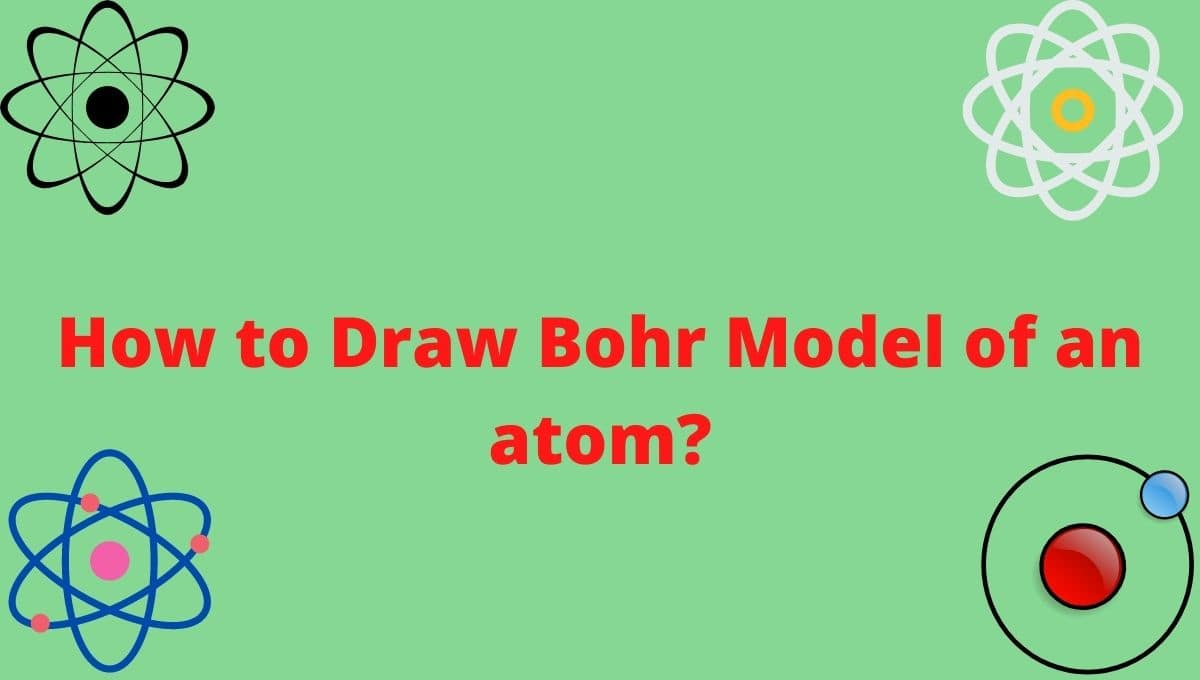Bohr model describes the visual representation of orbiting electrons around the small nucleus. It used different electron shells such as K, L, M, N…so on. These shells hold a specific number of electrons, the electron shell which is closest to the nucleus has less energy and the electron shell which is farthest from the nucleus has more energy.

In this article, we will study How to draw the Bohr model for an atom?

Bohr model or Rutherford–Bohr model, presented by Niels Bohr and Ernest Rutherford in 1913.

Bohr model of an atom consists of a small nucleus that contains protons and neutrons, this nucleus is surrounded by different electron shells or energy levels where electrons are revolved in a definite circular path “similar to the structure of the Solar System“.Protons are the positively charged particles and neutrons are the uncharged particles, both these are constituents of the atom nuclei. Electrons are negatively charged particles that orbit the nucleus of an atom.

“An electron shell may be thought of as an orbit followed by electrons around an atom’s nucleus”

Electron shells are also called the energy level, the electron shell located furthest from the nucleus region has more energy, and the electron shell(n=1) which is the closest to the nucleus region has less energy.

• Each electron shell holds a specific number of electrons and calculates by the 2n2 formula where n represents the shell number.
• The closest electron shell to the nucleus region is K-shell or 1st shell(n=1), hence, it can hold 2(1)2 = 2 maximum electrons.
• The 2nd shell(n=2) or L-shell is drawn after the 1st shell and can hold 2(2)2 = 8 maximum electrons.
• The 3rd shell(n=3) or M-shell holds 2(3)2 = 18 maximum electrons.
• The 4th shell(n=4) or N-shell holds 2(4)2 = 32 maximum electrons…..and so on.
Page Contents

## How to draw Bohr Model of an atom?

Bohr diagram is very interesting and easy to draw.

Let’s see How to draw a Bohr diagram for an atom? To draw the Bohr model of an atom, we should follow 4 or 5 basic steps.

1. Find the number of protons, electrons, and neutrons of an atom.
2. Draw the nucleus of an atom.
3. Write the number of protons and neutrons at the center of the nucleus.
4. Draw the first electron shell and put the electrons as a dot in it.
5. Draw the second electron shell, third electron shell….and so on, depending on the availability of the number of electrons.

Let’s take an example of the Fluorine atom to understand the “Concept of making Bohr model for an atom”.

## Steps to draw the Bohr Model of the atom

### 1. Find the number of protons, electrons, and neutrons

The number of protons for the atom is the same as its atomic number.

So, the atomic number for Fluorine is 9, hence, the number of protons in the Fluorine atom is also 9.

Now, to determine the number of neutrons in an atom, use this formula.

Number of neutrons in atom = Atomic mass of the atom(rounded to the nearest whole number) – Number of proton in an atom

Now, To get the number of neutrons in a Fluorine atom, look at its atomic mass which is 18.998 rounded to 19, and the number of protons in Fluorine is 9.

∴ Hence, the number of neutrons in Fluorine atom = (19 – 9) = 10 neutrons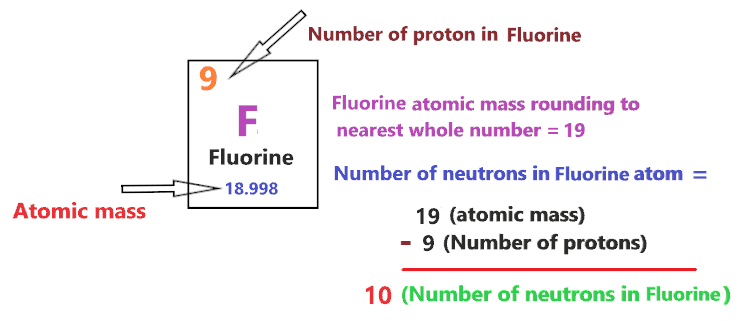It should be noted that “The number of electrons in a neutral atom is equal to the number of protons”.

So, the Fluorine atom is neutral, hence, its number of electrons will be equal to the number of protons which is Nine as we already discussed.

⇒ The number of electrons in Fluorine atom = 9

⇒ The number of protons in Fluorine atom = 9

⇒ The number of neutrons in Fluorine atom = 10

### 2. Draw the nucleus of an atom

In this step, we have to draw a small circle that consists of a number of protons and the number of neutrons of a Fluorine atom.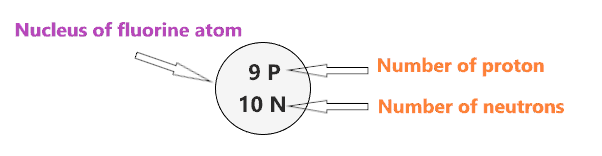### 3. Draw the First electron shell

The first electron shell is also called the K-shell, this is the closest shell to the nucleus of an atom and can hold a maximum of two electrons.

As we know, the Fluorine atom has a total of 9 electrons. So, put two electrons from it, in the first shell, next to each other.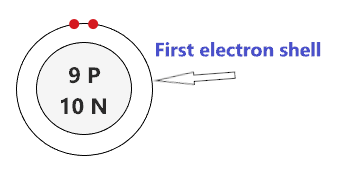As Fluorine atoms have a total of 9 electrons, and from 9 electrons we have used two electrons in the first shell.

∴ (9 – 2) = 7 electrons

Therefore, we are left with 7 electrons, let’s put them in the next shell of the fluorine atom.

### 4. Draw the Second electron shell

The second shell also called the L-shell that can hold a maximum of 8 electrons. This shell is drawn after the first electron shell.

Since we already used two electrons in the first shell, now, we have to put the remaining 7 electrons in the second shell.

In the second electron shell, the electrons are added one at a time, starting from the top position then going in a clockwise direction.

In second shell, electrons are added one at a time in clockwise direction as a clock position – 12 o’clock, 3 o’clock, 6 o’clock, 9 o’clock positions.Once you place the electrons one at a time to each of the four sides(Top – Right – Bottom – Left], start pairing or doubling them.

So, we have to put 7 remaining electrons of the Fluorine atom in the second shell, hence, start from the top position, put electrons one at a time, and go in a clockwise direction(Top – Right – Bottom – Left). And, finally, start pairing.That’s all, this is our Bohr model of the Fluorine atom that contains 9 protons and 10 neutrons in the nucleus region, and 9 electrons are orbited around the nucleus, two electrons in the first shell, and seven electrons in the second shell.

Let’s take another example and draw the Bohr model for the Phosphorus atom.

1. Find the number of protons, electrons, and neutrons

The atomic number for Phosphorus is 15, hence, the number of protons in the Phosphorus atom is also 15.

Now, To get the number of neutrons in a Phosphorus atom, look at its atomic mass which is 30.97 rounded to 31, and the number of protons in Phosphorus is 15.

∴ Hence, the number of neutrons in Phosphorus atom = (31 – 15) = 16 neutronsThe Phosphorus atom is neutral, hence, its number of electrons will be equal to the number of protons which is 15 as we already discussed.

⇒ The number of electrons in Phosphorus atom = 15

⇒ The number of protons in Phosphorus atom = 15

⇒ The number of neutrons in Phosphorus atom = 16

2. Draw the nucleus of an atom

In this step, we have to draw a small circle that consists of a number of protons and the number of neutrons of a Phosphorus atom.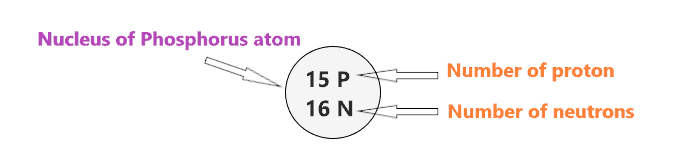3. Draw the First electron shell

As we know, the Phosphorus atom has a total of 15 electrons. So, put two electrons from it, in the first shell, next to each other.As a Phosphorus atom has a total of 15 electrons, and from 15 electrons we have used two electrons in the first shell.

∴ (15 – 2) = 13 electrons

Therefore, we are left with 13 electrons, let’s put them in the next shells of the Phosphorus atom.

4. Draw the Second electron shell

So, we have 13 remaining electrons of a Phosphorus atom, and the 2nd shell can only hold a maximum of 8 electrons.

Therefore, put the 8 electrons of the Phosphorus atom in the 2nd electron shell, start from the top position, put electrons one at a time, and go in a clockwise direction(Top – Right – Bottom – Left). And, finally, pair them up.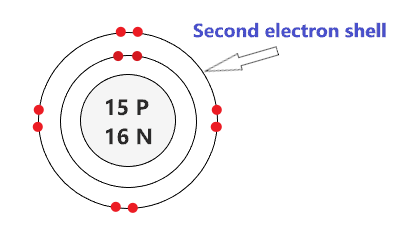As we have a total of 15 electrons for the Phosphorus atom and we placed 2 electrons in the first shell and 8 electrons in the second shell, hence, we are left with 5 electrons more.

Let’s place it in the next shell.

5. Draw the Third electron shell

The third shell is also called the M-shell and it has the capacity to hold up to 18 electrons but, for the elements in the first few periods, the third shell only holds up to 8 electrons.

“The third shell can be considered to hold 8 or 18 electrons but in total the third shell can hold 18 electrons.”

For the first few elements, say, till atomic number 20 on the periodic table, the third shell holds up to 8 electrons.

⇒ For atomic number 20 or less than 20, the third shell can hold up to maximum of 8 electrons.

⇒ For the atomic number more than 20, say, atomic numbers 21, 22, 23, 24, 25, 26, 27,…….etc., the third shell is filled with more than 8 electrons and up to maximum of 18 electrons.

For the Phosphorus atom, the third shell is filled with the same procedure as the second shell.

So, we have left with only 5 electrons of a Phosphorus atom, so, start from the top position, put electrons one at a time, and go in a clockwise direction(Top – Right – Bottom – Left). And, finally, start pairing.∴ This is our Bohr model of the Phosphorus atom that contains 15 protons and 16 neutrons in the nucleus region, and 15 electrons are orbited around the nucleus, two electrons in the first shell, eight electrons in the second shell, and five electrons in the third shell.

That’s all, this is How you can draw the Bohr-Rutherford diagram for atoms.

Now to get the complete detail for the Bohr model of various atoms, please go through the links given below.

## FAQ

### How to find the valence electron of an atom with the help of the Bohr model?

Valence electrons are found in the outermost shell of an atom and they can take participate in the formation of a chemical bond. These electrons have more energy compared to the inner shell electrons.

From the Bohr diagram of an atom, we can easily find the number of valence electrons in an atom by looking at its outermost shell.

Let’s take the example of the Neon Bohr model.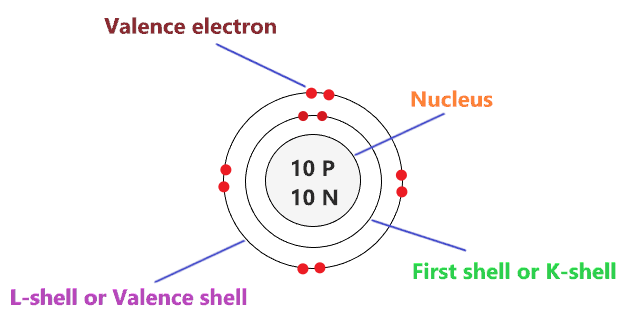Bohr’s diagram of Neon has only two electron shells (K and L), the inner shell is K-shell and the outermost shell is L-shell.

Hence, the electrons found in the L-shell of the Neon atom are its valence electrons because it is the outermost shell that also called the valence shell.

The L-shell or outer shell of the Neon Bohr model contains 8 electrons, therefore, the number of valence electrons in the Neon atom is 8.

### How to determine the number of electron shells a Bohr diagram contains?

Electron shell also called energy level, you can find the number of electron shells for an element by knowing its period number in the periodic table.

The elements or atoms in the first period of the periodic table have one energy level or one electron shell, same as, the elements in the second period have two energy levels or two-electron shells and so on…

First-period elements = One energy level

Second-period elements = Two energy level

Third-period elements = Three energy level

Fourth-period elements = Four energy level

## Summary

• How to Draw Bohr model of an atom? To draw the Bohr model diagram for any atom, first find the number of protons, electrons, and neutrons of the atom, then, draw the nucleus and write proton and neutrons at the center of it, after that, draw the first electron shell, second electron shell, third electron shell, etc. depending on the availability of electrons.
• The first shell of the Bohr-Rutherford diagram can hold only 2 electrons, the second shell can hold up to 8 electrons, the third shell can hold a maximum of 18 electrons, and the fourth shell can hold a maximum of 32 electrons.
• The electron shell of the Bohr model which is closest to the nucleus has less energy and the shell which is farthest away from the nucleus region have more energy.
• The farthest electron shell from the nucleus region of the Bohr diagram is also known as the Outermost shell which contains the valence electrons of an atom.
##### Subscribe to Blog via Email

Join 2 other subscribers

Share it...